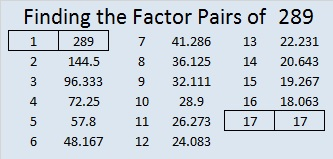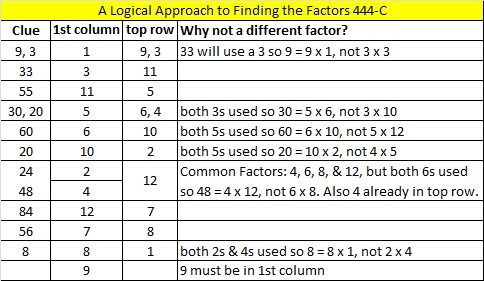# 289 and Level 6

• 289 is a composite number.
• Prime factorization: 289 = 17 x 17, which can be written 289 = (17^2)
• The exponent in the prime factorization is 2. Adding one we get (2 + 1) =  3. Therefore 289 has exactly 3 factors.
• Factors of 289: 1, 17, 289
• Factor pairs: 289 = 1 x 289 or 17 x 17
• 289 is a perfect square. √289 = 171 + 3 + 5 + 7 + 9 + 11 + … + 29 + 31 + 33 = 289

Teachfurthermaths is now including Find the Factors in its list of useful links!Print the puzzles or type the factors on this excel file: 12 Factors 2014-11-03